# Velarde solution gas oil ratio correlation

Jump to: navigation, search

## Velarde solution gas oil ratio correlation

Velarde solution gas oil ratio correlation is an empirical correlation for the solution gas oil ratio published in 1997. 

## Math & Physics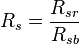$R_s = \frac{R_{sr}}{R_{sb}}$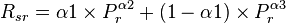$R_{sr} = \alpha1 \times P^{\alpha2}_r + (1 - \alpha1) \times P^{\alpha3}_r$

where: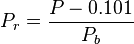$P_r = \frac{P-0.101}{P_b}$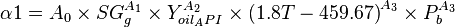$\alpha1 = A_0 \times SG^{A_1}_g \times Y^{A_2}_{oil_API} \times {(1.8 T- 459.67)}^{A_3} \times P^{A_3}_b$

A0 = 1.8653e-4
A1 = 1.672608
A2 = 0.929870
A3 = 0.247235
A4 = 1.056052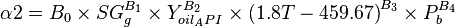$\alpha2 = B_0 \times SG^{B_1}_g \times Y^{B_2}_{oil_API} \times {(1.8 T - 459.67)}^{B_3} \times P^{B_4}_b$

B0 = 0.1004
B1 = -1.00475
B2 = 0.337711
B3 = 0.132795
B4 = 0.302065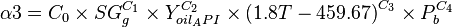$\alpha3 = C_0 \times SG^{C_1}_g \times Y^{C_2}_{oil_API} \times {(1.8 T - 459.67)}^{C_3} \times P^{C_4}_b$

C0 = 0.9167
C1 = -1.48548
C2 = -0.164741
C3 = -0.09133
C4 = 0.047094

## Example. Calculation of the solution gas oil ratio

Example source 

### Input data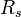$R_s$ = 60 sm3/sm3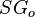$SG_o$ = 0.85 or 35 API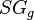$SG_g$ = 0.75$T$ = 90C or 363K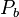$P_b$ = 11.65 MPa

Calculate solution gas oil ratio at p = 10 MPa?

### Solution

Pr = 0.8493
a1=0.1545
a2=1.8812
a3=0.5431
Rsr=0.8874
Rs=53.24 sm3/sm3

The solution is available in the online PVT calculator software at www.pengtools.com

## Application range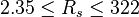$2.35 \le R_s \le 322$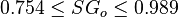$0.754 \le SG_o \le 0.989$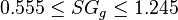$0.555 \le SG_g \le 1.245$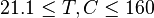$21.1 \le T, C \le 160$

## Nomenclature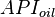$API_{oil}$ = oil specific gravity, °R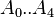$A_0..A_{4}$ = coefficients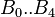$B_0..B_{4}$ = coefficients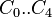$C_0..C_{4}$ = coefficients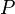$P$ = pressure, MPa$P_b$ = bubble point pressure, MPa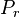$P_r$ = reduced pressure, MPa$R_s$ = solution oil gas ratio, sm3/sm3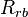$R_{rb}$ = solution oil gas ratio at bubble point pressure, sm3/sm3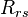$R_{rs}$ = reduced solution oil gas ratio, sm3/sm3$SG_g$ = gas specific gravity, dimensionless$SG_o$ = oil specific gravity, dimensionless$T$ = temperature, °K